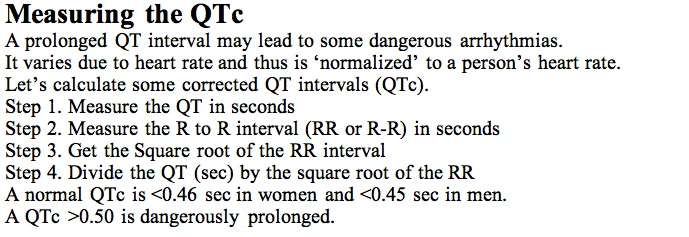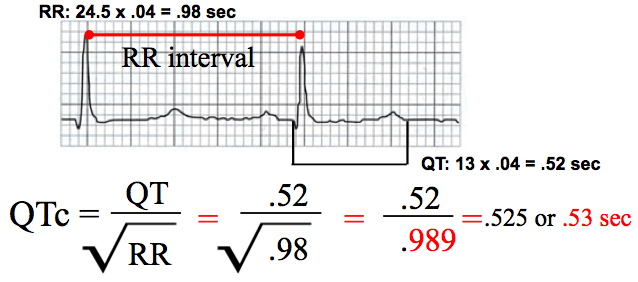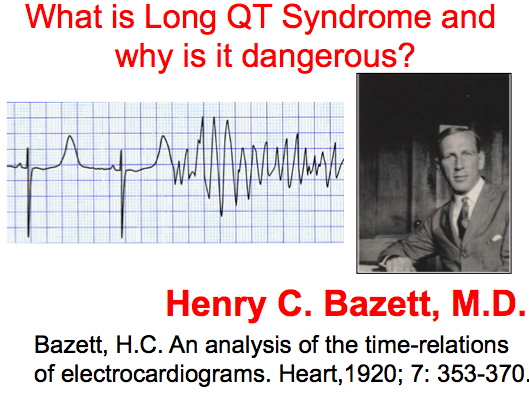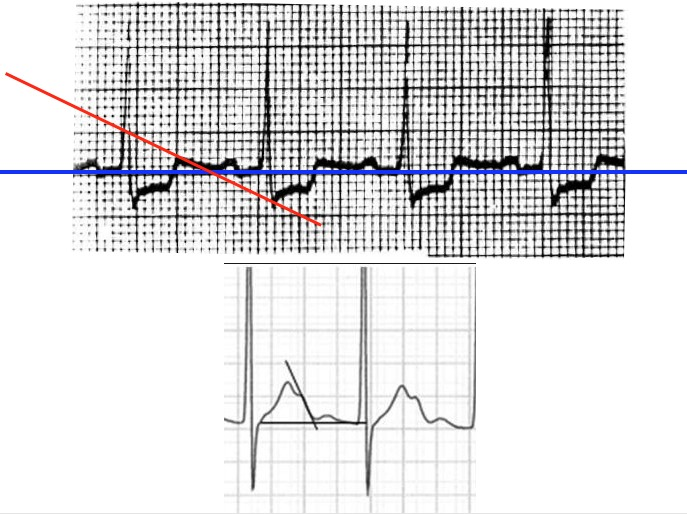Class, we use Bazett's Formula for the Calculation of QTc. See more on Dr. Bazett below.Here is how you calculate the QTcClass, take your RR Square root to THREE decimal places as shown (.989). Then, calculate your QTc and take it to TWO decimal places. If the 3rd significant figure of the QTc is '5' or higher please round up as above (QTc= .53 sec).Maximum Slope or Tangent methods of QT Interval measurementWhen it is difficult to tell where the QT interval ends, use the maximum slope (or tangent) technique. Draw a line using the PR segment that establishes a baseline on the EKG rhythm. Then draw a second line along the slope of the T wave. Where the drawn line intersects the isoelectric is the end of the QT interval.Top of Page | Research Interests | Vita | Articles | New Projects | Miscellaneous | UNM | Home
EKG Page